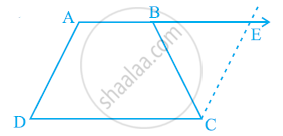# ABCD is a trapezium in which AB || CD and AD = BC (see the given figure). Show that - Mathematics

ABCD is a trapezium in which AB || CD and AD = BC (see the given figure). Show that

(i) ∠A = ∠B

(ii) ∠C = ∠D

(iv) diagonal AC = diagonal BD

[Hint: Extend AB and draw a line through C parallel to DA intersecting AB produced at E.]#### Solution

Let us extend AB. Then, draw a line through C, which is parallel to AD, intersecting AE at point E. It is clear that AECD is a parallelogram.

(i) AD = CE (Opposite sides of parallelogram AECD)

Therefore, BC = CE

∠CEB = ∠CBE (Angle opposite to equal sides are also equal)

Consider parallel lines AD and CE. AE is the transversal line for them.

∠A + ∠CEB = 180º (Angles on the same side of transversal)

∠A + ∠CBE = 180º (Using the relation∠CEB = ∠CBE) ... (1)

However, ∠B + ∠CBE = 180º (Linear pair angles) ... (2)

From equations (1) and (2), we obtain

∠A = ∠B

(ii) AB || CD

∠A + ∠D = 180º (Angles on the same side of the transversal)

Also, ∠C + ∠B = 180° (Angles on the same side of the transversal)

∴ ∠A + ∠D = ∠C + ∠B

However, ∠A = ∠B [Using the result obtained in (i)]

∴ ∠C = ∠D

AB = BA (Common side)

∠B = ∠A (Proved before)

∴ ΔABC ≅ ΔBAD (SAS congruence rule)

∴ AC = BD (By CPCT)

Concept: Another Condition for a Quadrilateral to Be a Parallelogram
Is there an error in this question or solution?
Chapter 8: Quadrilaterals - Exercise 8.1 [Page 147]

#### APPEARS IN

NCERT Class 9 Maths